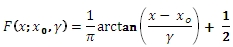# SQL Server Cauchy inverse distribution function

CAUCHYINV

Updated: 31 July 2010

Use CAUCHYINV to calculate the inverse lower cumulative distribution of the CAUCHY distribution.

The formula for the lower cumulative distribution function is:Syntax
SELECT [wctStatistics].[wct].[CAUCHYINV] (
<@P, float,>
,<@A, float,>
,<@B, float,>)
Arguments
@P
is the value of the lower cumulative distribution function. @P is an expression of type float or of a type that implicitly converts to float
@A
is the location parameter. @A is an expression of type float or of a type that implicitly converts to float
@B
is the shape parameter. @B is an expression of type float or of a type that implicitly converts to float
Return Types
float
Remarks
·         @B must be greater than zero (B > 0).
·         @P must be greater than or equal to zero and less than or equal to one (0 ≤ @P ≤ 1).
Examples
Calculate the inverse of the lower cumulative distribution function:
SELECT wct.CAUCHYINV(.75,0,1)
This produces the following result
----------------------
1

(1 row(s) affected)

### SupportCopyright 2008-2023 Westclintech LLC         Privacy Policy        Terms of Service